If you have a formula and want to show a specific result, but you do not know what input values to change within the formula, then Excel Goal Seek Formula is the one for you.

Excel Goal seek formula is an excellent way to minimize the efforts required in testing out a hit and trial method of finding answers. In a matter of seconds, it can find out the exact answer that would have taken a long time to find out manually.

It can easily be used for back calculations as well.

For example – You can set your net profit’s required amount to 0 in the Excel goal seek formula to find out the number of sales you have to generate to reach the breakeven point.

Similarly, any required amount to reach a certain goal can be calculated easily using the goal seek excel online.

The Goal Seel Formula comprises of three arguments, namely :

• Set cell – This is the cell that has the amount which we need to find out.
• To value – This is the value that you need the set cell amount to change to.
• By changing cell – This is the cell that will be changed to test out multiple possibilities and arrive at the exact amount.

Imagine you are calculating the payment terms on a loan.

Your PMT formula gives you an amount of \$1,450 but you can only afford to repay \$1,000. You can use Excel Goal Seek Formula to find out what Principal you can borrow based on your \$1,000 budget.

Watch it on YouTube and give it a thumbs-up!#### Follow the step-by-step tutorial on How to Use Excel Goal Seek Formula and download this Excel workbook to practice along:

STEP 1: Enter the 3 input variables that you will need to use for your PMT formula i.e. Interest Rate of 3.50%, Term of 240 months & Principal of \$250,000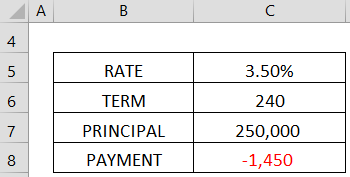STEP 2: Enter the PMT function in cell C8 =PMT(Interest Rate/12, Term, Principal) which will give you a monthly payment amount of -\$1,450.

Here using the PMT function, we have successfully linked the changing cell (principal cell) with the set cell (payment cell).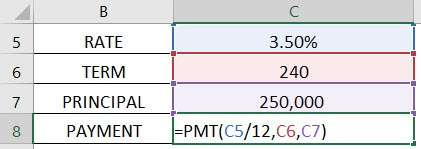STEP 3: Select the cell C8 and go to Data > What If Analysis > Goal Seek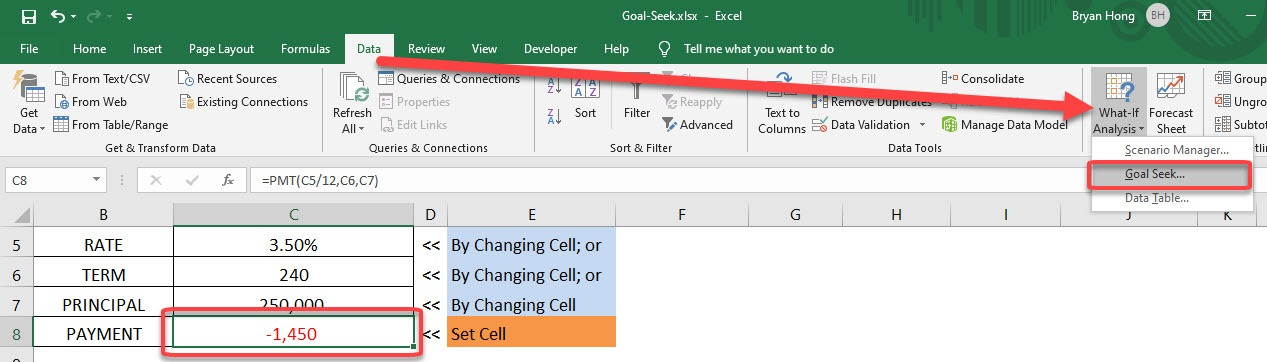STEP 4: SET CELL: Enter the reference for the cell that contains the formula that you want to resolve. In our example, this reference is cell C8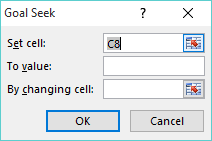STEP 5: TO VALUE: Type the formula result that you want.  In our example, we want the payment to be -\$1,000 (Note that this number is negative because it represents a payment)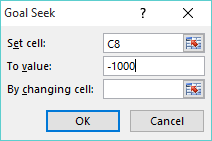STEP 6: BY CHANGING CELL: Enter the reference for the cell that contains the input value that you want to adjust i.e. One of our 3 variables (Interest Rate, Principal & Term).

In our example, this reference is cell C7 for the Principal.

It is important that this changing cell is linked directly or indirectly to the set cell. Otherwise, there will not be any possible way for excel to perform calculations if there is no link between the changing and the set cells.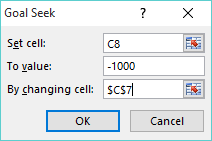STEP 7: Press OK and Excel Goal Seek Formula will run and produce a result.  Press OK to keep the results or Cancel to discard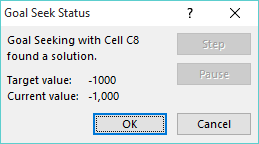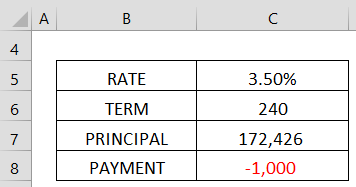Similarly, you can use this method to know how to use goal seek to find interest rate as well!

It may happen that Excel is not able to find an exact value via the goal seek function. It will get you the closest value and inform you that the goal-seeking may not have a solution.

In such cases, you can try the following methods to clarify this issue:

Method 1: Double-check goal seek parameters

While using Excel goal seek formula, it is essential that the required cell and the changing cells are either directly or indirectly dependent on each other.

There can be multiple layers of formulas that eventually link both the cells, but a link should be present for goal seek to work. Make sure that such a relationship exists between the two cells.

Method 2: Circular references

It should be noted that the changing cell and the required cell are not interdependent, i.e., there are no circular references present.

For Excel Goal Seek Formula to work properly, the involved formulas should not be co-dependent on each other.

Method 3: Adjusting the iteration settings

There’s a setting in Excel where you can adjust the number of possible solutions Excel will calculate, including changing its accuracy of the calculation.

To change them:

STEP 1: Click File from the tab list.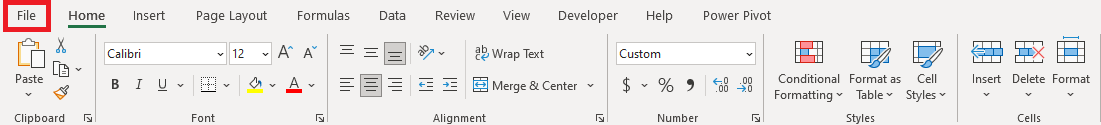STEP 2: Select Options.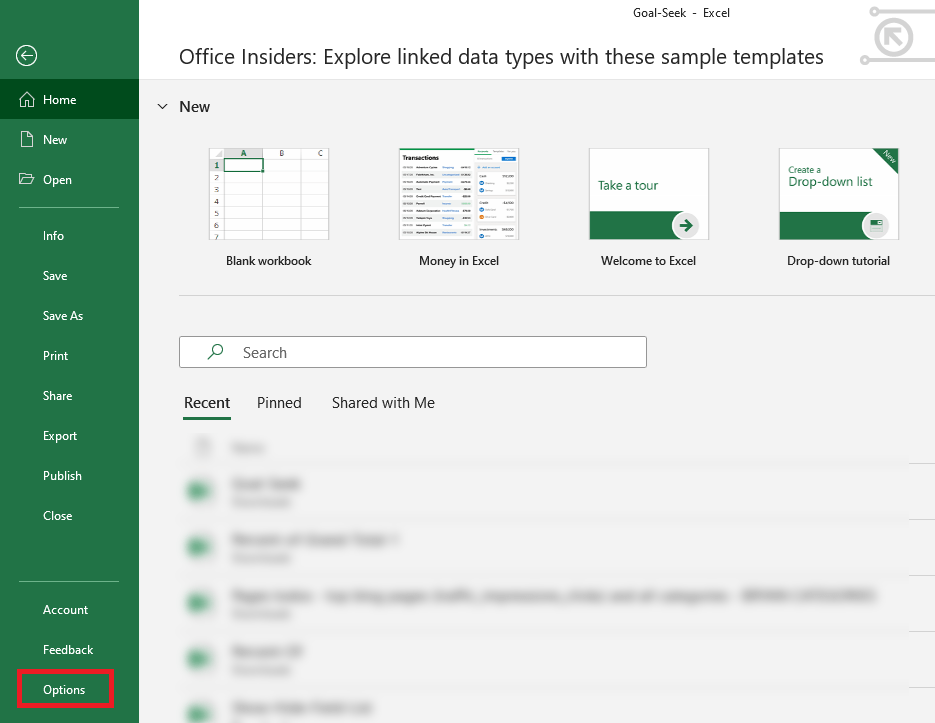STEP 3: Click on Formulas in the left vertical bar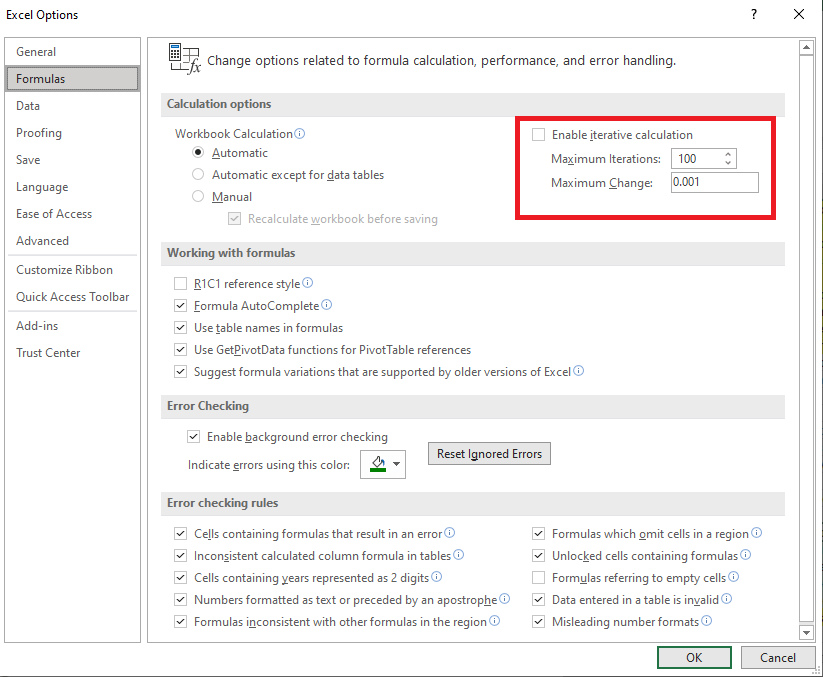Here, change these settings:

• Maximum Iterations (it denotes the number of possible solutions; the higher the number the more iterations). Increase this number if you want Excel to test out more possible solutions to the goal-seeking that you require.
• Maximum Change (it denotes the accuracy of the results; the lower the number the higher the accuracy). Decrease this number if your formula requires more accuracy. For example, if you are testing a formula with an input cell equal to 0 but Excel Goal Seek Formula stops at 0.001, setting Maximum Change to 0.0001 should fix the issue.